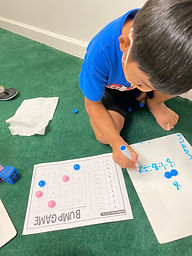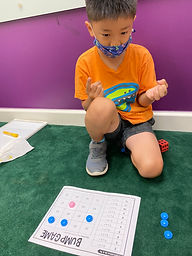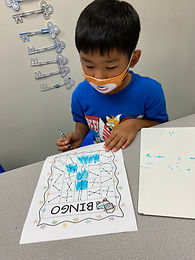## Ms. Brianne

### Target 1​

###### Lesson Type:

Continuation

Number Operation

:

Computation

Multiply within 100.

###### 1:

Understand that multiplying numbers makes numbers larger.

###### 2:

Understand that multiplication represents the combination of equal groups of objects.

###### 3:

Interpret products of whole numbers as the total number of objects arranged into equal groupings.

###### 4:

Understand that multiplication can be represented with arrays, repeated addition, and skip counting.

###### 5:

Understand that in multiplication equations, the first factor represents the number of groups and the second factor represents the quantity in each group.

3rd

###### Vocabulary:

Multiply, Times, Product, Equal Groups

Activities:

• Students solved multiplication problems using a strategy of their choice (skip counting, arrays, drawings, repeated addition).
• Students played a Bump game to practice multiplication problems. They used counting strategies, manipulatives or drew pictures to help them solve facts during the games.### Home Exploration

###### Guiding Questions:## Absent Students:

### Target 2

:

###### 1:

Understand that a geometric point is a fixed position in space.

###### 2:

Understand that a line contains an infinite number of points and has no end.

###### 3:

Understand that a line segment is a part of a line, containing two end points (so it has a beginning and an end).

###### 4:

Understand a ray is a combination of a line and a line segment, it has a start point with an endpoint and an arrow which indicates that it extends forever in only one direction.

4th

###### Vocabulary:

Point, Line, Segment, Ray, Parallel, Perpendicular

Activities:

• Students reviewed the different types of lines by drawing an example for each geometric term named.
• Students played a bingo game where they had to name and color in the type of line when given a description of its characteristics.
• Students created their own superhero, incorporating points, lines, line segments, and rays into their design.### Home Exploration

###### Guiding Questions:### Target 3

:

###### Vocabulary:

Activities:### Home Exploration